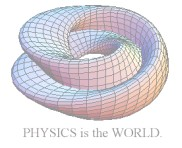[Japanese] [English]
I'm sorry, English page is only here in this site and my English is not well.
Plese tell me if you find my English mistakes. Thank you for your visit.
§Mechanics
 Simple Pendulum (java) a period of simple pendulum depends on amplitude. but it approximates to constant value in condition θ << 1. Double Pendulum (java) Simulate a double pendulum by using the Runge-Kutta method. Cycloidal Pendulum (java) Simulation of a cycloidal pendulum and its surroundings. Wilberforce Pendulum (Flash) About a Wilberforce Pendulum. sound New!! (java) You can beep your computer speaker using this applet.
§Electromagnetism
 Polarization (java) Simulation and explanation about polarization. electrification 1 (java) Simulate a lone charged sphere shell. electrification 2 (java) Simulate a charged sphere shell which is grounded. vector potential easy example of a vector potential. dielectric substances electric lines of force in boundary of dielectric substances. electric field (java) Simulate an electric field generated by point charges. You can set a charge on your demanding points. radiation Radiation from a harmonic oscillated charge. magnetic dipole potential from magnetic dipoles.
§Quantum Mechanics
 Schrödinger Equationon spherical coordinates How express Schödinger Eq. on spherical coordinates. Spherical Harmonics About Spherical Harmonics function.
§Statistical Mechanics
 2D Ising Model (java) Ising Model in 2D.
§Condensed Matter
 crystal structure 1 (java) Simulate the experiments determine the lattice constant from a X-ray picture took by the powder method.
§Physical Mathematics
 Lissajous (java) Drawing the Lissajous curve which is compound from the harmonic oscillators crossed perpendicular. Koch Lines. (java) Drawing the Koch lines gradually. Taylor expansion fundamental explanation of the taylor expansion. Fourier seriesrenew! fundamental explanation of the Fourier series.
§tools
 linealize (javascript) calculate a linear line from (x,y) datas. probably error (javascript) calculate probably errors from n datas log scale (javascript) Transform datas from (x,y) to logscale (x',y')